﻿ Golden Ratio. Fibonacci Number Stock Vector - Illustration of dynamic, division: 143897854

# Golden ratio. Fibonacci number.

## Golden ratio. Fibonacci number. Ideal proportions. Geometric shape. Mathematical concepts. Abstract background. Vector illustration

More similar stock illustrations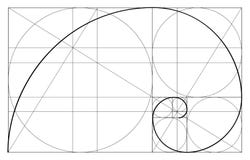Minimalistic style design. Golden ratio. Geometric shapes. Circles in golden proportion. Futuristic design. Logo. Vector icon. Abs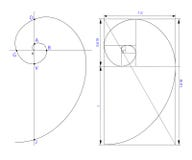Golden ratio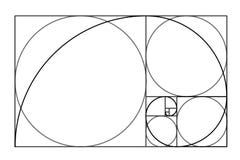Minimalistic style design. Golden ratio. Geometric shapes. Circles in golden proportion. Futuristic design. Logo. Vector iconSpiral golden section Golden ratio proportion Fibonacci spiral icon set white color vector illustration flat style image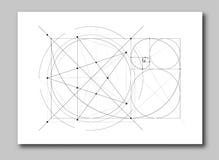Golden ratio section abstract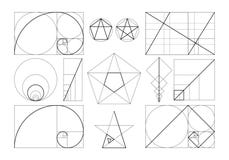Template of figures in frame of golden ratio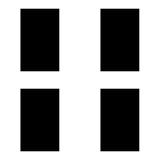Vertical black rectangles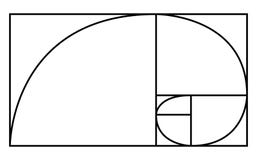Golden Ratio icon or logo in modern line style.Black simple spiral logo, sign, symbol, vector, icon,
More stock photos from Archivector's portfolio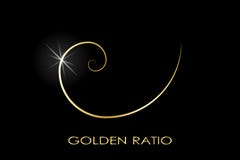Golden ratio. Logo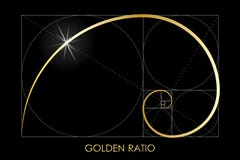Golden ratio. Harmonic division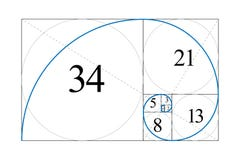Golden ratio. Fibonacci number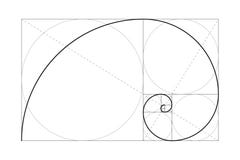Golden ratio. Drawing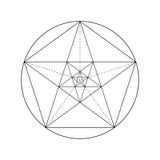Pentagram star drawing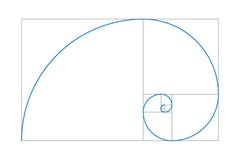Golden section drawing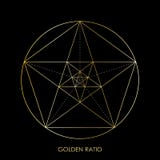Pentagram star. Golden ratio
Related categories